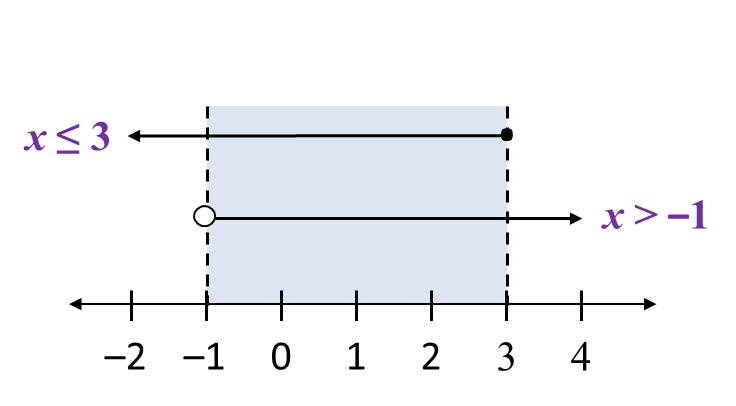# 7.1.2 Linear Inequalities

7.1.4 Solve Inequalities in One Variable
To solve linear inequalities in one variable, use inverse operation to make the variable as the subject of the inequality.

Example:
Solve the following linear inequalities.

Solution:
(a)

(b)

7.1.5 Simultaneous Linear Inequalities in One Variable
1.   The common values of two simultaneous inequalities are values which satisfy both linear inequalities.The common values of the simultaneous linear inequalities x ≤ 3 and x > –1 is –1 < x ≤ 3.

2.   To solve two simultaneous linear inequalities is to find a single equivalent inequality which satisfies both inequalities.